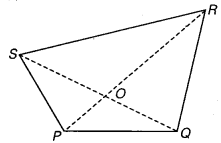# Class 6 Maths NCERT Solutions for Chapter 4 Basic Geometrical Ideas Ex – 4.5

## Basic Geometrical Ideas

Question 1.
Draw a rough sketch of a quadrilateral PQRS. Draw its diagonals. Name them. Is the meeting point of the diagonals in the interior or exterior of the quadrilateral?

Solution:
PQRS is a quadrilateral having PR and QS as its diagonals intersecting at the point O, which is in the interior of the quadrilateral PQRS.Question 2.
Draw a rough sketch of a quadrilateral KLMN. State,

(a) two pairs of opposite sides,
(b) two pairs of opposite angles,
(c) two pairs of adjacent sides,
(d) two pairs of adjacent angles.

Solution:

(a)
KL, MN and LM, KN are two pairs of its opposite sides.
(b) ∠K, ∠M and∠L, ∠N are two pairs of opposite angles.
(c) KL, LM and KN, NM are two pairs of adjacent sides.
(d) ∠K, ∠N and ∠L, ∠M are two pairs of adjacent angles.

Question 3.
Investigate:

Use strips and fasteners to make a triangle and a quadrilateral.
Try to push inward at any one vertex of the triangle. Do the same to the quadrilateral.
Is the triangle distorted? Is the quadrilateral distorted? Is the triangle rigid?
Why is it that structures like electric towers make use of triangular shapes and not quadrilaterals?

Solution:
On pushing inward at any one vertex of the triangle, we find that the triangle is not distorted. Whereas doing so with the quadrilateral we find that it is distorted. Triangle is rigid. Thus, we make use of triangular shapes in structures like electric towers as triangular shapes are more rigid.